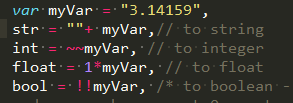# 我们要不要在 JS 使用二进制位运算？

Javascript 完全套用了 Java 的位运算符，包括按位与`&`、按位或`|`、按位异或`^`、按位非`~`、左移`<<`、带符号的右移`>>`和用0补足的右移`>>>`

## 到底会慢多少？

`````` javascript

console.time('*2');
for (var i = 0; i < 10000000; i++) {
1.1 * 2
}
console.timeEnd('*2');

console.time('<<1');
for (var i = 0; i < 10000000; i++) {
1.1 << 1
}
console.timeEnd('<<1');

console.time('/4');
for (var i = 0; i < 10000000; i++) {
1.1 / 4
}
console.timeEnd('/4');

console.time('>>2');
for (var i = 0; i < 10000000; i++) {
1.1 >> 2
}
console.timeEnd('>>2');

``````

``````
*2: 29.870ms
<<1: 33.389ms
/4: 33.003ms
>>2: 27.841ms
``````

`````` javascript
console.time('| 0');
for (var i = 0; i < 10000000; i++) {
i / 3 | 0
}
console.timeEnd('| 0');

console.time('Math.floor');
for (var i = 0; i < 10000000; i++) {
Math.floor(i / 3)
}
console.timeEnd('Math.floor');

console.time('parseInt(x)');
for (var i = 0; i < 10000000; i++) {
parseInt(i / 3)
}
console.timeEnd('parseInt(x)');

console.time('parseInt(x, 10)');
for (var i = 0; i < 10000000; i++) {
parseInt(i / 3, 10)
}
console.timeEnd('parseInt(x, 10)');

console.time('(| 0) + 1');
for (var i = 0; i < 10000000; i++) {
(i / 3 | 0) + 1
}
console.timeEnd('(| 0) + 1');

console.time('Math.ceil');
for (var i = 0; i < 10000000; i++) {
Math.ceil(i / 3)
}
console.timeEnd('Math.ceil');
``````

`````` javascript
| 0: 55.003ms
Math.floor: 55.196ms
parseInt(x): 197.627ms
parseInt(x, 10): 131.175ms
(| 0) + 1: 28.960ms
Math.ceil: 113.976ms
``````

`````` javascript
Math.ceil('1234.23'); //1235
parseInt('1234,23', 10); //1234
``````

## JS 使用二进制位运算的一些例子

| 运算符 | 用法 | 描述 | | --- | --- | --- | | 按位与（AND） | `a & b` | 对于每一个比特位，只有两个操作数相应的比特位都是1时，结果才为1，否则为0。 | | 按位或（OR） | `a \| b` | 对于每一个比特位，当两个操作数相应的比特位至少有一个1时，结果为1，否则为0。 | | 按位异或（XOR） | `a ^ b` | 对于每一个比特位，当两个操作数相应的比特位有且只有一个1时，结果为1，否则为0。 | | 按位非（NOT） | `~ a` | 反转操作数的比特位，即0变成1，1变成0。 | | 左移（Left shift） | `a << b` | 将 a 的二进制形式向左移 b (< 32) 比特位，右边用0填充。 | | 有符号右移 | `a >> b` | 将 a 的二进制表示向右移 b (< 32) 位，丢弃被移出的位。 | | 无符号右移 | `a >>> b` | 将 a 的二进制表示向右移 b (< 32) 位，丢弃被移出的位，并使用 0 在左侧填充。 |

### 随机获取数组某项

`````` javascript
var arr = [...];
var len = arr.length;

// 一般方法：
var rIndex = Math.floor((Math.random() * len))

// 使用位运算的方法
var rIndex = Math.random() * len | 0
``````

### 让`indexOf()`返回`true`or`false`

`````` javascript
var arr = [...];

// 一般方法：
var isExist = function(array, value) {
return array.indexOf(value) > 0;
}

// 使用位运算的方法：将 -1 转变为 false
var isExist = function(array, value) {
return !!~array.indexOf(value);
}
``````

#### 补充：ES6/7 和`indexOf()`类似的 API

`````` javascript
// .find()
[1, 5, 10, 15].find(function(value, index, arr) {
return value > 9;
}) // 10，未找到的话，返回 undefined

// .findIndex()
[1, 5, 10, 15].findIndex(function(value, index, arr) {
return value > 9;
}) // 2，未找到的话，返回 -1

// .includes()
[1, 2, 3].includes(2);     // true
[1, 2, 3].includes(4);     // false
[1, 2, NaN].includes(NaN); // true
``````

### 切换变量 0 或 1

`````` javascript
// 一般方法：
if (toggle) {
toggle = 0;
} else {
toggle = 1;
}

// 一般方法的简写：
togle = toggle ? 0 : 1;

// 使用位运算的方法：
toggle ^= 1;
``````

### 碰撞检测优化（标示我需不要和你检测碰撞）

| | Bullet | Tank | Wall | | --- | --- | --- | --- | | impactFlag | 1 | 2 | 4 | | impactFlags | 2+4 | 0 | 0 |

`````` javascript
doCheckImpact = obj1.impactFlag & obj2.impactFlags // 非0标示需要进行碰撞检测（0 == false）
``````

``````
7 = 111

1 = 001
2 = 010
4 = 100
``````

`````` javascript
// 以前的版本
function leftpad (str, len, ch) {
str = String(str);

var i = -1;

if (!ch && ch !== 0) ch = " ";

len = len - str.length;

while (++i < len) {
str = ch + str;
}

return str;
}
``````

`````` javascript
// 左耳朵耗子的 PR
function leftpad (str, len, ch) {
//convert the `str` to String
str = str +'';

len = len - str.length;
if (len <= 0) return str;

//convert the `ch` to String
if (!ch && ch !== 0) ch = ' ';
ch = ch + '';

while (true) {
if (len & 1) pad += ch;
len >>= 1;
if (len) ch += ch;
else break;
}
}
``````

`len`和 1 去按位与是为了看右移后的`len`是奇数还是偶数，比如：

`````` javascript
2 & 1 //0
3 & 1 //1
4 & 1 //0
5 & 1 //1
``````

`````` javascript
// 月影大神的
str = "" + str;
if(!ch && ch !== 0) ch = " ";
ch = "" + ch;

var padlen = len - str.length;

ch += ch;
}
}
}
``````#### 补充 ES6/7 相关字符串拓展

##### .repeat()

`repeat`方法返回一个新字符串，表示将原字符串重复n次。

`````` javascript
'x'.repeat(3) // "xxx"
'hello'.repeat(2) // "hellohello"
'na'.repeat(0) // ""
``````

ES7推出了字符串补全长度的功能。如果某个字符串不够指定长度，会在头部或尾部补全。`padStart`用于头部补全，`padEnd`用于尾部补全。

`````` javascript

``````

## 最后

• 点赞
• 写回答
• 关注问题
• 收藏
• 复制链接分享
• 邀请回答

#### 6条回答

• 常用的~~array = [myVar]; // to array

点赞 评论 复制链接分享
• http://www.basecss.net/2014/03/27/code-learning/ by

点赞 评论 复制链接分享
• *2: 41.327ms <<1: 28.248ms 我的是chrome 50，结果是这样，多次执行偶有移位比直接乘慢的情况

点赞 评论 复制链接分享
• 嗯，确实有，其实我觉得对于简单本身可以用直接运算的比必要用位运算，但是我们没有必要排斥它。

点赞 评论 复制链接分享
• 习惯用 `~~` 取整

点赞 评论 复制链接分享
• 恩 两次按位非 负负得正

点赞 评论 复制链接分享